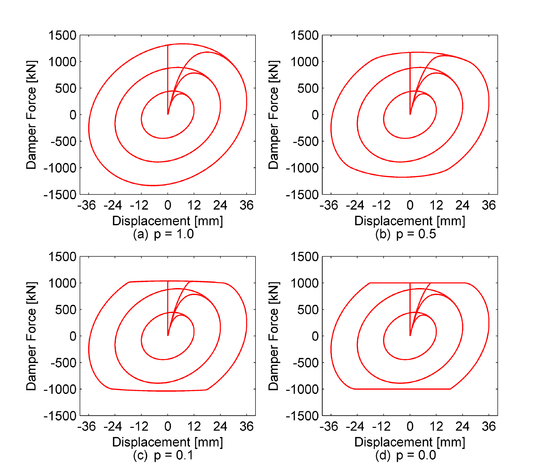# BilinearOilDamper Material

Jump to navigationJump to search

This command is used to construct a BilinearOilDamper material, which simulates the hysteretic response of bilinear oil dampers with relief valve. Two adaptive iterative algorithms have been implemented and validated to solve numerically the constitutive equations within a bilinear oil damper with a high-precision accuracy.

 uniaxialMaterial BilinearOilDamper \$matTag \$K \$Cd <\$Fr \$p> <\$LGap> < \$NM \$RelTol \$AbsTol \$MaxHalf>

 \$matTag integer tag identifying material \$K Elastic stiffness of linear spring to model the axial flexibility of an oil damper (brace and damper portion) \$Cd Viscous damping coefficient of an oil damper (before relief) \$Fr Damper relief load (default=1.0, Damper property) \$p Post-relief viscous damping coefficient ratio (default=1.0, linear oil damper) \$LGap gap length to simulate the gap length due to the pin tolerance (default=0.0: zero tolerance) \$NM Employed adaptive numerical algorithm (default value NM = 1; 1 = Dormand-Prince54, 2=adaptive finite difference) \$RelTol Tolerance for absolute relative error control of the adaptive iterative algorithm (default value 10^-6) \$AbsTol Tolerance for absolute error control of adaptive iterative algorithm (default value 10^-10) \$MaxHalf Maximum number of sub-step iterations within an integration step (default value 15)

Examples:

 1. Input parameters: Assume a bilinear oil damper with axial stiffness K=200.0kN/mm, viscous damping coefficient C=6.0KN/(mm/s), relief load Fr=1000.0KN, p=0.1. The input parameters for the material should be as follows: uniaxialMaterial BilinearOilDamper 1 200.0 6.0 1000 0.1 Using these properties, Figure 1c shows the hysteretic response of this damper for sinusoidal displacement increments of 12, 24 and 36mm and a frequency f = 1.0Hz. Figures 1a-1d show the damper hysteresis with varying post-relief viscous damping coefficient ratio (p=1.0, 0.5, 0.1, 0.0).Figure 1.Oil Damper with various post-relief viscous damping coefficient ratios Assume a bilinear oil damper with axial stiffness K=200.0kN/mm, viscous damping coefficient C=6.0KN/(mm/s), relief load Fr=1000.0KN, p=0.1 and LGap = 0.5mm due to the pin tolerance at the damper ends. The input parameters for the material should be as follows: uniaxialMaterial BilinearOilDamper 1 200.0 6.0 1000 0.1 0.5 Using these properties, Figure 2c shows the hysteretic response of this damper for sinusoidal displacement increments of 0.5, 1 and 1.5mm and a frequency f = 1.0Hz. Figures 2a-2d show the damper hysteresis with varying gap length (LGap = 0.0, 0.2. 0.5. 1.0 mm)Figure 2.Oil Damper with various gap lengths

References:

  Akcelyan, S., Lignos, D. G., Hikino, T. (2018). “Adaptive Numerical Method Algorithms for Nonlinear Viscous and Bilinear Oil Damper Models Subjected to Dynamic Loading.” Soil Dynamics and Earthquake Engineering, 113, 488-502. .  Akcelyan, S. (2017). "Seismic retrofit of existing steel tall buildings with supplemental damping devices." Ph.D. Dissertation, McGill University, Canada.

Code Developed and Implemented by : Sarven Akcelyan & Prof. Dimitrios G. Lignos, (McGill University)